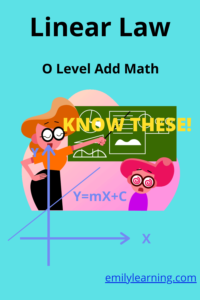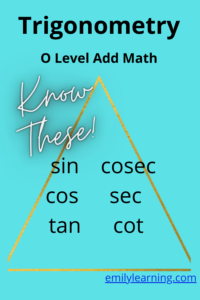# Category: O Level

Here, you will find all the resources for O Level exams. We cover O level Chemistry, Physics and Additional Mathematics .## What is tested for linear law tested in O Level Additional Mathematics

Let’s talk about what you need to know about linear law in O Level Additional Mathematics. Basically, in this topic, you are required to convert…## What you need to know for Polynomials and Partial Fractions tested in O Level Additional Mathematics

Polynomials (including factor and remainder theorem and cubic equations) and partial fractions are easy to score for O Level Additional Mathematics, if you know the…## What you need to know for coordinate geometry, circles and proofs in plane geometry tested in O Level Additional Mathematics

Let’s talk about what you need to know for Coordinate geometry, circles (in coordinate geometry) and proofs in plane geometry for O Level Additional Mathematics.…## What you need to know for binomial expansion tested in O Level Additional Mathematics

For binomial expansion for O Level Additional Mathematics, you are tested only on binomial expansions with powers that are positive integers. Meaning if you have…## What you need to know for Trigonometry for O Level Additional Mathematics

Trigonometry is a big topic in O Level Additional Mathematics. There are many different concepts in this topic Trigonometry.  For Additional Math, students need to…## What you need to know for O Level for Surds, Indices and Logarithms (O Level Add Maths)

Surds, indices, and logarithms are chapters that are tested in O Level Additional Mathematics. You also need to apply what you have learnt in these…## What you need to know for O Level for Quadratic Functions (O Level Add Maths)

In O Level Additional Mathematics, this is what you need to know for quadratic functions: Finding maximum and minimum of a quadratic function by completing…Here, you will find the suggested solutions to the O Level Add Math Specimen Paper 2. The specimen paper can be downloaded from the Singapore…